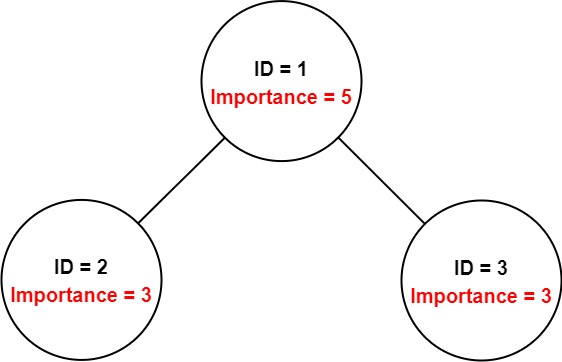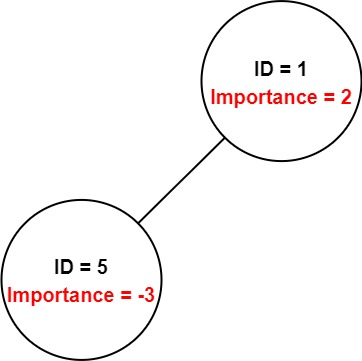## Algorithm

Problem Name: 690. Employee Importance

You have a data structure of employee information, including the employee's unique ID, importance value, and direct subordinates' IDs.

You are given an array of employees `employees` where:

• `employees[i].id` is the ID of the `ith` employee.
• `employees[i].importance` is the importance value of the `ith` employee.
• `employees[i].subordinates` is a list of the IDs of the direct subordinates of the `ith` employee.

Given an integer `id` that represents an employee's ID, return the total importance value of this employee and all their direct and indirect subordinates.

Example 1:```Input: employees = [[1,5,[2,3]],[2,3,[]],[3,3,[]]], id = 1
Output: 11
Explanation: Employee 1 has an importance value of 5 and has two direct subordinates: employee 2 and employee 3.
They both have an importance value of 3.
Thus, the total importance value of employee 1 is 5 + 3 + 3 = 11.
```

Example 2:```Input: employees = [[1,2,],[5,-3,[]]], id = 5
Output: -3
Explanation: Employee 5 has an importance value of -3 and has no direct subordinates.
Thus, the total importance value of employee 5 is -3.
```

Constraints:

• `1 <= employees.length <= 2000`
• `1 <= employees[i].id <= 2000`
• All `employees[i].id` are unique.
• `-100 <= employees[i].importance <= 100`
• One employee has at most one direct leader and may have several subordinates.
• The IDs in `employees[i].subordinates` are valid IDs.

## Code Examples

### #1 Code Example with C++ Programming

```Code - C++ Programming```

``````
// BFS
class Solution {
public:
int getImportance(vector employees, int id) {
unordered_mapm;
for(auto x: employees) m[x->id] = x;
int sum = 0;
dequeq;
q.push_back(m[id]);
while(!q.empty()){
auto p = q.front();
q.pop_front();
for(auto x: p->subordinates) q.push_back(m[x]);
sum += p->importance;
}
return sum;
}
};

// DFS
class Solution {
public:
int getImportance(vector employees, int id) {
unordered_mapm;
for(auto x: employees) m[x->id] = x;
int sum = 0;
DFS(m, id, sum);
return sum;
}

void DFS(unordered_map& m, int id, int& sum){
sum += m[id]->importance;
for(auto x: m[id]->subordinates) DFS(m, x, sum);
}
};
``````
Copy The Code &

Input

cmd
employees = [[1,5,[2,3]],[2,3,[]],[3,3,[]]], id = 1

Output

cmd
11

### #2 Code Example with Javascript Programming

```Code - Javascript Programming```

``````
const GetImportance = function (employees, id) {
const map = {}
employees.forEach((employee) => {
map[employee.id] = employee
})
const s = [id]
let importance = 0
while (s.length) {
let current = map[s.pop()]
importance += current.importance
if (current.subordinates.length) {
s.push(...current.subordinates.reverse())
}
}
return importance
}
``````
Copy The Code &

Input

cmd
employees = [[1,5,[2,3]],[2,3,[]],[3,3,[]]], id = 1

Output

cmd
11

### #3 Code Example with Python Programming

```Code - Python Programming```

``````
class Solution:
def getImportance(self, employees, id):
"""
:type employees: Employee
:type id: int
:rtype: int
"""
def dfs(id):
self.val += dic[id].importance
for sub in dic[id].subordinates:
dfs(sub)
dic = {}
for emp in employees:
dic[emp.id] = emp
self.val = 0
dfs(id)
return self.val
``````
Copy The Code &

Input

cmd
employees = [[1,2,],[5,-3,[]]], id = 5

Output

cmd
-3

### #4 Code Example with C# Programming

```Code - C# Programming```

``````
using System.Collections.Generic;
using System.Linq;

namespace LeetCode
{

public class _0690_EmployeeImportance
{
public int GetImportance(IList employees, int id)
{
var map = employees.ToDictionary(e => e.id, e => e);
return DFS(map, id);
}

private int DFS(IDictionary map, int id)
{
var result = map[id].importance;
foreach (var subId in map[id].subordinates)
result += DFS(map, subId);
return result;
}

public class Employee
{
public int id;
public int importance;
public IList subordinates;
}
}
}
``````
Copy The Code &

Input

cmd
employees = [[1,2,],[5,-3,[]]], id = 5

Output

cmd
-3